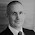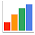## Tuesday, July 15

### Seasonal adjustment and decomposition

Having moved from R to python/pandas, one of the things I really miss is the extensive statistics library available in R.

I particularly like the STL function: the seasonal decomposition of a time series by Loess (localised regression). The function allows you to decompose a time series into its seasonal, trend and irregular components using loess. Unfortunately, I could find nothing like it in pandas.

In frustration, I have cobbled together a python/pandas function to decompose a univariate pandas time series item into seasonal, trend and irregular components. The function does not use Loess. Rather it uses a set of processes similar to those used by the Australian Bureau of Statistics.

It also uses a Henderson Moving Average which I had coded previously (see here).

Anyway, I'd be interested to know whether this code works for you. Drop me a line if you end up using it.

Caution: still experimental code.

```## Decompose.py
## produce a time series decomposition

import pandas as pd
import numpy as np
from Henderson import Henderson

# --- A selection of seasonal smoothing weights, from which you can select
#     Note: these are end-weights, they are reversed for the start of a series
#     Note: your own weights in this form should also work
s3x3 = (
np.array([ 5, 11, 11]) / 27.0,
np.array([ 3,  7, 10,  7]) / 27.0,
np.array([ 1,  2,  3,  2,  1]) / 9.0,
)

s3x5 = (
np.array([ 9, 17, 17, 17]) / 60.0,
np.array([ 4, 11, 15, 15, 15]) / 60.0,
np.array([ 4,  8, 13, 13, 13,  9]) / 60.0,
np.array([ 1,  2,  3,  3,  3,  2,  1]) / 15.0,
)

s3x9 = (
np.array([0.051, 0.112, 0.173, 0.197, 0.221, 0.246]),
np.array([0.028, 0.092, 0.144, 0.160, 0.176, 0.192, 0.208]),
np.array([0.032, 0.079, 0.123, 0.133, 0.143, 0.154, 0.163, 0.173]),
np.array([0.034, 0.075, 0.113, 0.117, 0.123, 0.128, 0.132, 0.137, 0.141]),
np.array([0.034, 0.073, 0.111, 0.113, 0.114, 0.116, 0.117, 0.118, 0.120, 0.084]),
np.array([1,     2,     3,     3,     3,    3,    3,    3,    3,    2,    1]) / 27.0,
)

# --- public Decomposition function
def Decompose(s, periods=None, model='multiplicative',
constantSeasonal=False, seasonalSmoother=s3x5):

""" The simple decomposition of a pandas Series s into its trend, seasonal
and irregular components. The default is a multiplicative model:
Original(t) = Trend(t) * Seasonal(t) * Irregular(t). Can specify an
additive model: Original(t) = Trend(t) + Seasonal(t) + Irregular(t)

Parameters:
-   s - the pandas Series, without any missing or NA values,
and sorted in ascending order
-   periods - either a pandas Series indicating the period to
which each value of s belongs (of the same length as s,
with the same index as s), or an int for the number of periods
into which to decompose the series
-   model - string - either 'multiplicative' or 'additive'
-   constantSeasonal - bool - whether the seasonal component is
constant or (slowly) variable
-   seasonalSmoother - when not using a constantSeasonal, which
of the seasonal smoothers to use (s3x3, s3x5 or s3x9) -
default is s3x5 (ie over 7 years for monthly or quarterly data)

Returns a pandas DataFrame with columns for each step in the
decomposition process (enables debugging). The key columns in the
DataFrame are:
-   'Original' - the original series
-   'SeasAdj' - the seasonally adjusted series
-   'Trend' - the trend of the seasonally adjusted series
-   'Seasonal' - the seasonal component found through the
decomposition process
-   'Irregular' - the irregular component found through the
decomposition process

Notes:
Based on ideas gleaned from the Australian Bureau of Statistics:
ABS (2005), "An Introductory Course on Times Series
Analysis -- Electronic Delivery", Catalogue: 1346,0.55.001, online at:
http://www.ausstats.abs.gov.au/ausstats/free.nsf/0/C2714BAD1DD803E6CA256F960072B0C0/\$File/1346055001_jan%202005.pdf
Does not adjust for moving holidays, public holidays, variable number
of working days in month, etc. (ie. it is quite a simple decomposition)"""

### --- Sanity checks and initialisation --- ###

# --- sanity checks
if periods is None:
raise ValueError('The periods parameter is an integer or a Series of integers')
if not isinstance(s, pd.core.frame.Series):
raise TypeError('The s parameter should be a pandas Series')
if not(s.index.is_monotonic and s.index.is_unique):
raise ValueError('The index for the s parameter should be unique and sorted')
if any(s.isnull()) or not all(np.isfinite(s)):
raise ValueError('The s parameter contains NA or infinite values')

# --- initialise
result = pd.DataFrame(s)
result.columns = ['Original']

# --- determine the period
if isinstance(periods, pd.core.frame.Series):
if not (len(s) == len(periods) and all(s.index == periods.index)) :
raise ValueError('The s and periods parameters must have the same index')
result['period'] = periods
periods = len(periods.unique())
else:
periods = int(periods)
result['period'] = pd.Series(range(len(result)), index=s.index) % periods
if periods < 2:
raise ValueError('The periods parameter should be >= 2')
if len(s) < (periods * 2) + 1:
raise ValueError('The s parameter is not long enough to decompose')

# --- settle the length of the Henderson moving average
h = max(periods, 7) # ABS uses 13-term HMA for monthly and 7-term for quarterly
if h % 2 == 0 :
h += 1 # we need an odd number

### --- On to the decomposition process --- ###

# --- 1 - derive an initial estimate for the trend component
result['1stTrendEst'] = pd.rolling_mean(s, window=periods+1,
min_periods=periods+1, center=True)
# Note: rolling mean leaves NA values at the start/end of the trend estimate.

# --- 2 - preliminary estimate of the seasonal component
if model == 'multiplicative':
result['1stSeasonalEst'] = result['Original'] / result['1stTrendEst']
else:
result['1stSeasonalEst'] = result['Original'] - result['1stTrendEst']

# --- 3 - smooth the seasonal
result = _smoothSeasonalComponent(result, periods=periods,
constantSeasonal=constantSeasonal, seasonalSmoother=seasonalSmoother,
columnToBeSmoothed='1stSeasonalEst', newColumn='2ndSeasonalEst')

# --- 4 - extend the smoothed seasonal estimate to full scale
if any(result['2ndSeasonalEst'].isnull()) :
result = _extendSeries(result, periods=periods,
columnToBeExtended='2ndSeasonalEst', newColumn='3rdSeasonalEst')
else:
result['3rdSeasonalEst'] = result['2ndSeasonalEst']

# --- 5 - preliminary estimate of the seasonally adjusted data
if model == 'multiplicative':
result['1stSeasAdjEst'] = result['Original'] / result['3rdSeasonalEst']
else:
result['1stSeasAdjEst'] = result['Original'] - result['3rdSeasonalEst']

# --- 6 - a better estimate of the trend
result['2ndTrendEst'] =  Henderson(result['1stSeasAdjEst'], h)

# --- 7 - final estimate of the seasonal component
if model == 'multiplicative':
result['4thSeasonalEst'] = result['Original'] / result['2ndTrendEst']
else:
result['4thSeasonalEst'] = result['Original'] - result['2ndTrendEst']

result = _smoothSeasonalComponent(result, periods=periods,
constantSeasonal=constantSeasonal, seasonalSmoother=seasonalSmoother,
columnToBeSmoothed='4thSeasonalEst', newColumn='Seasonal')

# --- 8 - final estimate of the seasonally adjusted series
if model == 'multiplicative':
result['SeasAdj'] = result['Original'] / result['Seasonal']
else:
result['SeasAdj'] = result['Original'] - result['Seasonal']

# --- 9 - final trend estimate
result['Trend'] =  Henderson(result['SeasAdj'], h)

# --- 10 - final irregular
if model == 'multiplicative':
result['Irregular'] = result['SeasAdj'] / result['Trend']
else:
result['Irregular'] = result['SeasAdj'] - result['Trend']

# --- 11 - our job here is done
return (result)

# --- apply seasonal smoother
def _smoothSeasonalComponent(result, periods, constantSeasonal, seasonalSmoother,
columnToBeSmoothed, newColumn):

# get the key smoothing constants
if not constantSeasonal:
kS = len(seasonalSmoother)
lenS = (len(seasonalSmoother) * 2) -1
centralS = seasonalSmoother[len(seasonalSmoother)-1]

# establish an empty return column ...
result[newColumn] = np.repeat(np.nan, len(result))

# populate the return column ...
for u in result['period'].unique() :

# get each of of the seasonals
thisSeason = result[result['period'] == u][columnToBeSmoothed]

# smooth to a constant seasonal value
if constantSeasonal:
thisSeasonSmoothed = pd.Series(np.repeat(thisSeason.mean(skipna=True),
len(thisSeason)), index=thisSeason.index)

# smooth to a slowly changing seasonal value
else:
# drop NA values which result from step 1 in the decomp process
thisSeason = thisSeason.dropna()

# apply the seasonalSmoother
thisSeasonSmoothed = pd.rolling_apply(thisSeason, window=lenS,
func=lambda x: (x * centralS).sum(), min_periods=lenS, center=True)

# for short series the above process results in no data ... find a simple mean
if all(thisSeasonSmoothed.isnull()) :
# same treatment as constant seasonal value above
thisSeasonSmoothed = pd.Series(np.repeat(thisSeason.mean(skipna=True),
len(thisSeason)), index=thisSeason.index)

# handle the end-point problem ...
for i in range(kS-1) :
if np.isnan(thisSeasonSmoothed.iat[i]) :
thisSeasonSmoothed.iat[i] = (thisSeason.iloc[0: i+kS] *
(seasonalSmoother[i][::-1])).sum() # note: reversed order at start

for i in range(len(thisSeason)-1, len(thisSeason)-kS, -1) :
if np.isnan(thisSeasonSmoothed.iat[i]) :
thisSeasonSmoothed.iat[i] = (
thisSeason.iloc[i-(kS-1):len(thisSeason)] *
seasonalSmoother[len(thisSeason)-1-i]).sum()

# package up season by season ...
result[newColumn] = result[newColumn].where(result[newColumn].notnull(),
other=thisSeasonSmoothed)

return (result)

# --- extend seasonal components to the full length of series
def _extendSeries(result, periods, columnToBeExtended, newColumn):

result[newColumn] = result[columnToBeExtended].copy()

def fillup(result, fill, startPoint, endPoint):
i = startPoint
while True:
p = result.index[i]
result[newColumn].iat[i] = fill[newColumn].at[result['period'].iat[i]]
if p >= endPoint:
break
i += 1

# back-cast
if np.isnan(result.iat[0, result.columns.get_loc(newColumn)]):
fill = pd.DataFrame(result[newColumn].dropna().iloc[0:periods])
fill['period'] = result['period'][fill.index:fill.index[len(fill)-1]]
endPoint = fill.index - 1
fill.index = fill['period']
fillup(result=result, fill=fill, startPoint=0, endPoint=endPoint)

# forward-cast
if np.isnan(result.iat[len(result)-1, result.columns.get_loc(newColumn)]):
fill = result[newColumn].dropna()
fill = pd.DataFrame(fill[len(fill)-periods:len(fill)])
fill['period'] = result['period'][fill.index:fill.index[len(fill)-1]]
startPoint = result.index.get_loc(fill.index[len(fill)-1] + 1)
fill.index = fill['period']
endPoint = result.index[len(result)-1]
fillup(result=result, fill=fill, startPoint=startPoint, endPoint=endPoint)

return (result)
```

#### 2 comments:

1.Not my area - but some of your posts have given me some insight for python, so:

Did you look at Andreas Hiboll's python wrapper for the R's STL, which also uses Pandas (https://gist.github.com/andreas-h/7808564)

Or his python only version (https://github.com/andreas-h/pyloess)

1.Thanks for the heads-up - I was not aware of these.